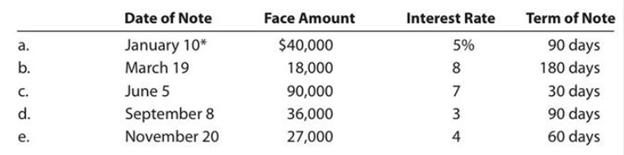Chapter 9, Problem 9.19EX

Chapter
Section
Textbook Problem

Determine due date and interest on notesDetermine the due date and the amount of interest due at maturity on the following notes:*Assume that February has 28 days.

To determine

Note receivable:

Note receivable refers to a written promise received by the creditor from the debtor in formal, for the amounts to be settled within a stipulated period of time. This written promise is issued by a debtor or borrower to the lender or creditor. Notes receivable is an asset of a business. Notes receivable often used for the credit periods of more than 60 days.

Due date:

Due date is the maturity date on note, on due date the borrower is supposed to repay the face value of the note along with interest.

Interest on note:

Interest on note is the amount charged on the principal value of note for the privilege of borrowing money. Interest is to be paid by the borrower and to be received by the lender.

To determine: The due date and the amount of interest due at maturity.

Explanation

Determine the due date and the amount of interest due at maturity.

 Due date Amount of interest due at maturity a. April 10 $500 b. September 15$720 c. July 5 $525 d. December 7$270 e. January 19 $180 Table (1) Working note: a. Determine the due date for the note issued on April 10, whose term period is 90 days. The due date of the note is calculated as follows:  Particulars Days Number of days from January 10 to 31 22 days Number of days from February 1 to 28 28 days Number of days from March 1 to 30 30 days Number of days from April 1 to 10 10 days Total 90 days Table (2) Calculate the amount of interest due at maturity. Total interest=[Face amount ×Annual interest rate×Time in terms of year]=[$40,000×5%100×90 days360 days]=$500 b. Determine the due date for the note issued on March 19, whose term period is 180 days. The due date of the note is calculated as follows:  Particulars Days Number of days from March 20 to 31 12 days Number of days from April 1 to 30 30 days Number of days from May 1 to 31 31 days Number of days from June 1 to 30 30 days Number of days from July 1 to 31 31 days Number of days from August 1 to 31 31 days Number of days from September 1 to 15 8 days Total 180 days Table (3) Calculate the amount of interest due at maturity. Total interest=[Face amount ×Annual interest rate×Time in terms of year]=[$18,000×8%100×180 days360 days]=\$720

c.

Determine the due date for the note issued on June 5, whose term period is 30 days.

The due date of the note is calculated as follows:

 Particulars Days Number of days from June 6 to 30 25 days Number of days from July 1 to 5 5 days Total 30 days

Table (4)

Calculate the amount of interest due at maturity

Still sussing out bartleby?

Check out a sample textbook solution.

See a sample solution

The Solution to Your Study Problems

Bartleby provides explanations to thousands of textbook problems written by our experts, many with advanced degrees!

Get Started

Find more solutions based on key concepts